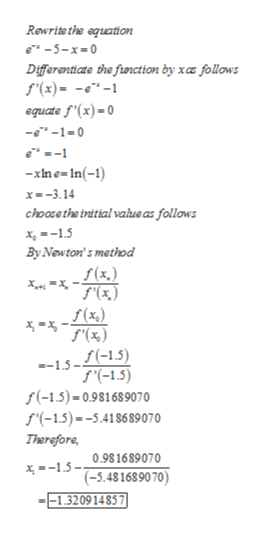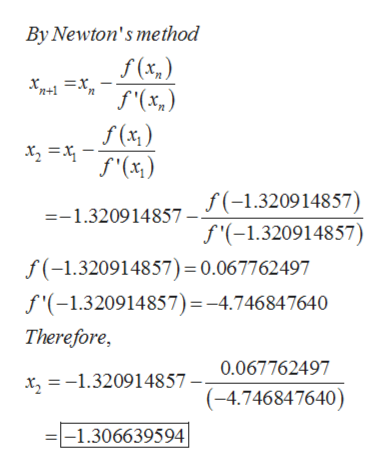# Use newtons method to approximate a root of the equation e^(-x) = 5 + x correct to eight decimal places.

Question
36 views

Use newtons method to approximate a root of the equation e^(-x) = 5 + x correct to eight decimal places.

check_circle

Step 1

Use newtons method to approximate a root of the equation e(-x) = 5 + x correct to eight decimal places.

Step 2

Consider the equation

e-x=5+xhelp_outlineImage TranscriptioncloseRewrite the aquation -5-x-0 Differanticate the fiunction by xaz follows f'(x)= --1 equate f'(x)=0 --1-0 xine-In(-1) x-3.14 choose the irnitial value as follows x15 By Newton's method f(x.) f (x,) f'(%) 1.5--15) f(-1.5) f(-1.5)-0.981689070 f(-1.5)--5.418689070 Therefore 0.981689070 x--15- (-5.481689070) --1.320914857 fullscreen
Step 3

At n=1

...help_outlineImage TranscriptioncloseBy Newton's method f(x,) 2 f'(x) f(-1.320914857) f'(-1.320914857) =-1.320914857 f(-1.320914857) = 0.067762497 f'(-1.320914857) = -4.746847640 Therefore, 0.067762497 x -1.320914857 - (-4.746847640) -1.306639594 fullscreen

### Want to see the full answer?

See Solution

#### Want to see this answer and more?

Solutions are written by subject experts who are available 24/7. Questions are typically answered within 1 hour.*

See Solution
*Response times may vary by subject and question.
Tagged in

### Calculus